# Applying Noetherianness

This is a survey article about applying the concept/definition/theorem: Noetherianness
View other application survey articles OR view survey articles related to Noetherianness

This is a survey article on how to apply the condition that a given commutative unital ring is a Noetherian ring. The notion of Noetherianness is an important generalization of a number of the features common between a multivariate polynomial ring over a field and a ring of integers in a number field.

Although Noetherianness is a crucial assumption for giving geometric interpretation to various aspects of a ring's structure, it is not a very strong assumption. A number of Noetherian rings exhibit non-geometric behavior.

## Change of base ring and going to modules

Often, we are given that a particular base ring is Noetherian. We use certain facts, described below, to deduce that certain other rings are Noetherian.

### Noetherianness is closed under taking affine rings

Further information: Noetherianness is polynomial-closed, Noetherianness is quotient-closed

If$A$ is a Noetherian ring, then$A[x]$ is a Noetherian ring for any$x$. By inducting on this, we see that$A[x_1,x_2,\ldots,x_n]$ is again Noetherian.

It's also true that any quotient of a Noetherian ring is quotient. Combining these two facts, we see that any algebra that is finitely generated as an algebra over a Noetherian ring is again a Noetherian ring, because any finitely generated algebra is a quotient of a polynomial ring over the base ring.

This is useful in a number of ways. For instance:

• Any finitely generated algebra over a field (often called an affine ring) is Noetherian. This means that all the rings coming up in algebraic geometry in this fashion, are Noetherian.
• All finite extensions of Noetherian rings are Noetherian. Thus, any ring that is a degree$d$ extension of a Noetherian ring, is Noetherian. Thus, any ring of integers in a number field is Noetherian.

It is also crucial in jacking up the base ring, for instance, in the following proofs:

• Artin-Tate lemma: Here, the crucial idea is to change the base to a larger ring which is finitely generated over the Noetherian base, and such that the ring that we're targeting, is finitely generated over it.
• Artin-Rees lemma: Here, the crucial idea is to pass from a given Noetherian ring to its blowup algebra at some ideal, and use the fact that that blowup algebra is again Noetherian. This has two steps: observe that since the ring is Noetherian, the ideal is a finitely generated ideal and hence the blowup algebra is finitely generated. We then use the fact that a finitely generated algebra over a Noetherian ring is Noetherian.

### Localization and its effects

Further information: Noetherianness is localization-closed

If$R$ is a Noetherian ring, the localization of$R$ at any multiplicatively closed subset (and in particular, the localization with respect to prime or maximal ideals) is a Noetherian local ring.

### Completion and its effects

Fill this in later

### Passing to modules

A module over a ring is termed a Noetherian module if every submodule of it is finitely generated. The set of Noetherian modules over a ring is closed under taking finite direct sums, submodules, and quotients. Thus, if a ring is itself Noetherian, any finitely generated module over it is a Noetherian module. In particular:

Over a Noetherian ring, any submodule of a finitely generated module is finitely generated

This is an alternative description of Noetherianness, and is sometimes the more useful one. For instance:

• Artin-Tate lemma: Here, the main fact we use is this: we find a ring and a finitely generated module over it, and argue that therefore, a certain submodule of it is also finite.

## Constructing ascending chains

One application of Noetherianness is to construct ascending chains of ideals, and argue that they stabilize. The general process is:

• Use the given data to construct an ascending chain of ideals
• Observe that Noetherianness forces the chain to stabilize
• Use the stabilization of the chain to solve the problem

Often, what we have are not chains, but more complicated collections.

Also, we may sometimes, instead of looking at an ascending chain of ideals, look at an ascending chain of submodules in a finitely generated module. Since any finitely generated module is Noetherian, the same argument works.

### The tree approach

This is an illustration of the principle of Noetherianness. Call an ideal in a Noetherian ring an irreducible ideal if it cannot be expressed as the intersection of two strictly bigger ideals. Then, it is true that every ideal in a Noetherian ring is expressible as an intersection of finitely many irreducible ideals.

To prove this, we construct a rooted binary tree. We start with the given ideal. If it is irreducible, stop. Otherwise, express it as an intersection of two bigger ideals. Make the two bigger ideals its two children. Then continue the process with the two children. Proceed like this to construct a tree.

We need to use Noetherianness to argue that the tree has finite depth, since that would give us a finite intersection. In other words, we need to argue that the tree does not have paths of arbitrarily large lengths. A short combinatorial argument, called Konig's lemma, states that if the tree has paths of arbitrarily large lengths, then it has a path of infinite length. However, a path of infinite length would yield a strictly ascending infinite chain of ideals: a contradiction.

The key step here is the use of Konig's lemma, which is not immediately obvious and requires a proof by contradiction.

Ideas analogous to the tree approach are used in the following proofs:

• Primary decomposition theorem which combines this with the fact that in a Noetherian ring, any irreducible ideal is primary
• The topological result that any Noetherian space is a union of finitely many irreducible subspaces

### The maximum condition

The same results as the tree approach can be achieved if, instead of using the tree, we use a maximum condition. Essentially, this provides an alternative to the combinatorial Konig's lemma. The maximum condition states that any collection of ideals in a Noetherian ring has maximal elements; more specifically, every element is contained inside a maximal element.

### Using quotients

A superb approach to constructing ascending chains is that of using quotients. Quotients convert a descending chain to an ascending chain. Thus, if we have a descending chain of ideals:$I_1 \supset I_2 \supset I_3 \supset \ldots$

and$Q$ is a fixed ideal, then: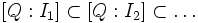$[Q:I_1] \subset [Q:I_2] \subset \ldots$

is an ascending chain of ideals. Here$[Q:J] = \{ x : Jx \subset Q \}$. Thus, even though we have no reason to suppose that the original descending chain stabilizes, the ascending chain will.

A particular case of this is when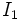$I_1$ is a principal ideal. Then the ascending chain is: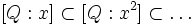$[Q:x] \subset [Q:x^2] \subset \ldots$

This ascending chain is used to prove that in a Noetherian ring, any irreducible ideal is primary.

### Directed sets

A partially ordered set$I$ is a directed set if for any two elements, there is an element at least as big as both of them. The maximum condition on ideals tells us the following: given a directed set of ideals, there is a unique maximal element, and this is the union of all the ideals. This can also be seen by a direct argument using ascending chains.

Here is an example where we apply this. Suppose$S$ is a multiplicatively closed subset of a ring. Then, given an ideal$Q$, the set of ideals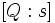$[Q:s]$, where$s \in S$, form a directed set under inclusion. That's because given$s_1, s_2 \in S$,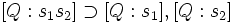$[Q:s_1s_2] \supset [Q:s_1], [Q:s_2]$. Hence, Noetherianness tells us that we can find an element$s$ such that$[Q:s]$ is a union of all such ideals.

An adaptation of this, to the case of modules, is used to prove that localization respects associated primes for Noetherian rings.